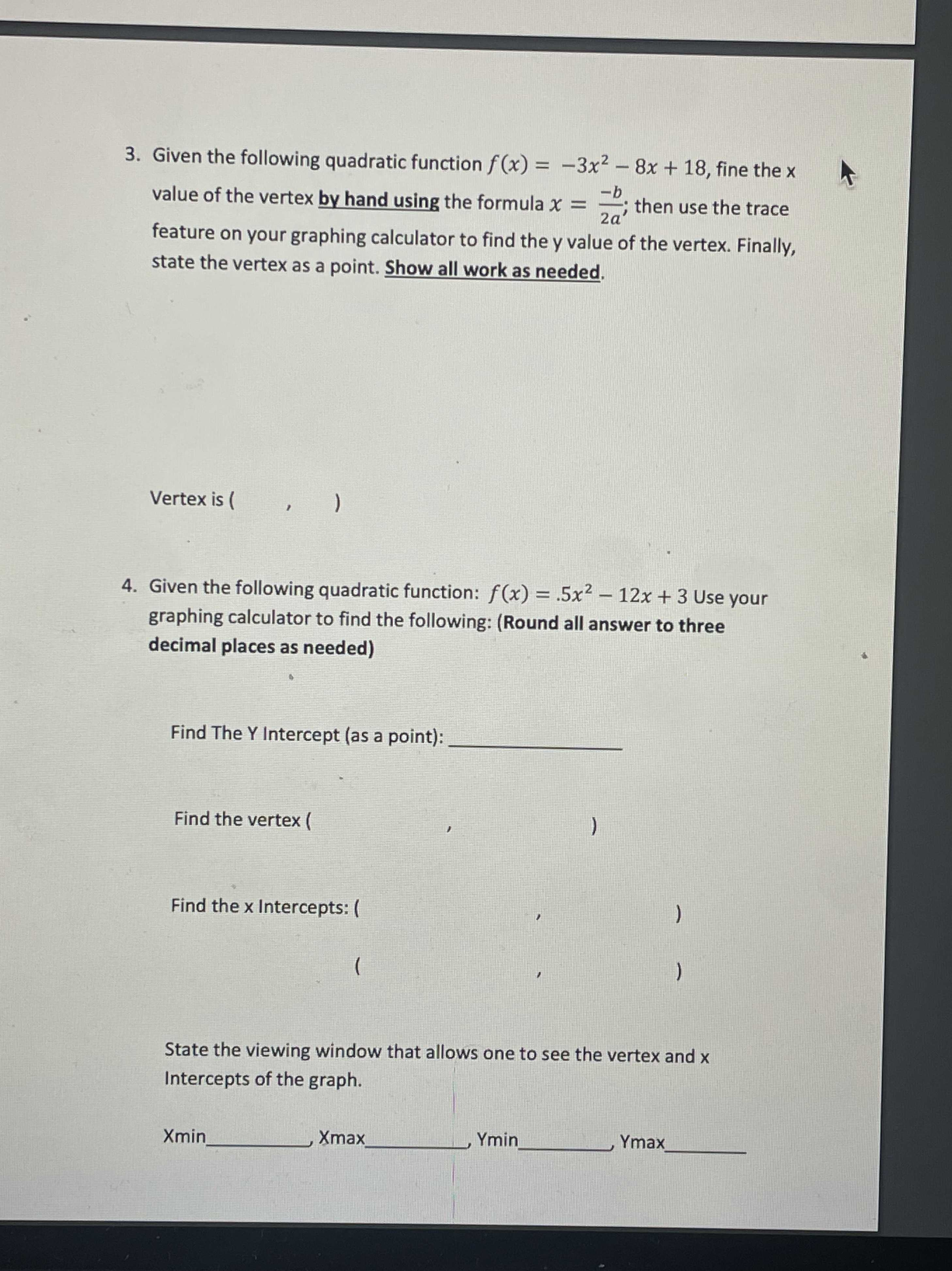### ¿Todavía tienes preguntas de matemáticas?

Pregunte a nuestros tutores expertos
Algebra
Pregunta3. Given the following quadratic function $$f ( x ) = - 3 x ^ { 2 } - 8 x + 18$$ , fine the $$x$$ value of the vertex by hand using the formula $$x = \frac { - b } { 2 a }$$ ; then use the trace feature on your graphing calculator to find the $$y$$ value of the vertex. Finally, state the vertex as a point. Show all work as needed.

Vertex is ( )

4. Given the following quadratic function: $$f ( x ) = .5 x ^ { 2 } - 12 x + 3$$ Use your graphing calculator to find the following: (Round all answer to three decimal places as needed)

Find The Y Intercept (as a point):

Find the vertex

Find the $$x$$ Intercepts:

State the viewing window that allows one to see the vertex and $$x$$ Intercepts of the graph. Xmin Xmax Ymin Ymax

3. x=-b/2a= -(-8)/2(-3)= -4/3

y(-4/3)= -3(-4/3)^2 -8(-4/3)+18= 70/3

vertex is (-4/3, 70/3)

4. y intercept: (0,3)

vertex: (12,-69)

x intercepts: (23.747,0),(0.253,0)

Xmin -4/3, Ymin 70/3

Solución
View full explanation on CameraMath App.